﻿ Models for Forecasting the Demand and Supply of Electricity in Nigeria

### Models for Forecasting the Demand and Supply of Electricity in NigeriaOPEN ACCESSPEER-REVIEWED

## Models for Forecasting the Demand and Supply of Electricity in Nigeria

Benjamin Oyediran Oyelami1,, Adedamola adedoyin Adewumi2

1Theoretical Physics, National Mathematical Centre, Abuja, Nigeria

2Department of Mathematics, University of Abuja, Nigeria

### Abstract

In this paper, the Garch model is used to determine the market volatility in the demand and supply chains of electricity in Nigeria for 36 years, i.e. from 1970 to 2005 from the historic data obtained from the National Bureau of Statistics. The Harvey logistic model is used to predict the demand and supply of electricity in the country from 2005 to 2026. From the results obtained, the demand and supply of electricity show mixture of spikes because of mean reverse behavior about the mean values and the demand outweighed the supply. The consequence of this is that there is vast market prosperity for investors in the power sector in Nigeria. It is estimated that about 317.5 billion US dollars business opportunities are available to new independent power stations in Nigeria.

### At a glance: Figures

1234
Prev Next

• Oyelami, Benjamin Oyediran, and Adedamola adedoyin Adewumi. "Models for Forecasting the Demand and Supply of Electricity in Nigeria." American Journal of Modeling and Optimization 2.1 (2014): 25-33.
• Oyelami, B. O. , & Adewumi, A. A. (2014). Models for Forecasting the Demand and Supply of Electricity in Nigeria. American Journal of Modeling and Optimization, 2(1), 25-33.
• Oyelami, Benjamin Oyediran, and Adedamola adedoyin Adewumi. "Models for Forecasting the Demand and Supply of Electricity in Nigeria." American Journal of Modeling and Optimization 2, no. 1 (2014): 25-33.

 Import into BibTeX Import into EndNote Import into RefMan Import into RefWorks

### 1. Introduction

Electricity power supply is the most important commodity for the development of a nation. People become easily empowered to work and develop themselves when there is electricity power supply. This development takes place from domestic level to industrial level and can also be transferred from small to medium and to large scale level. Power consumption demand depends on the population and industrialization of a country [2, 4, 5, 15]. There is power equilibrium when the supply is equal to the demand, otherwise there is supply shortfall.

Models for pricing, forecasting and supply of electricity are often characterized by spikes. Electricity is non-storable, hence the market is volatility and will change with time, and that is, the volatility has heteroscedasicity behavior which is a kind of time varying variance [13, 14]. There are several researches done on modeling and forecasting the demand and supply of electricity. Oyelami  studied the dynamics of demand and supply of electricity pricing and Barlow’s model was used to suggest that capping happen at some maximum price whenever demand exceeds the maximum supply.

Electricity supply in Nigeria dates back to 1886 when two small generating sets were installed to serve the then colony of Lagos. By an act of parliament in 1951, the Electricity Corporation of Nigeria (ECN) was established and in 1962, the Niger Dams Authority (NDA) was also established. However, a merger of the two was made in 1972 to form the National Electric Power Authority (NEPA) as a result of unbundling and power reform process, it was renamed Power Holding Company of Nigeria (PHCN) in 2005 . In February 2005, the World Bank agreed to provide PHCN with \$100 million to assist in its privatization efforts. In November 2013, the Federal government of Nigeria (FGN) concluded the privatization of power sector in Nigeria which led to selling of the PHCN to some privately owned companies.

Nigeria is a country blessed with a lot of resources such as coal, oil, natural gas, water and other renewable energy sources that can be used to generate electricity. The renewable energy being solar energy, biomass, wind, small and large hydropower with potential for hydrogen fuel, geothermal and ocean energies. Nigeria is Africa’s major oil producing country which accounts for two-thirds of Africa’s crude oil reserves. Talking about Nigerian natural gas reserves, it is estimated to be 187.44 trillion standard cubic feet in 2005 . These reserves are known to be substantially larger than its oil reserves in terms of energy. Gas utilization for generation of electricity is said to be a primary goal for Nigeria. The first discovery of coal was in the year 1909; the mining began in 1916 with a recorded output of about 24,500 tons . The poor industrialization and varying economic growth in Nigeria may be attributed to unreliable and inadequate power supply.

The socio-economic development activities in the country are responsible for the increase in demand for electricity. Mostly, this high demand can be found in the manufacturing and commercial sub-sectors. The problem of Nigeria appears to be lower electricity supply compared to the electricity consumption.

As at 2007, Nigeria has about 14 generating plants with an installed capacity of 7,876MW but the installed available capacity is less than 4000MW as at 2009. Nigerian electricity supply market has a lot of potential profit to investors if they invest in the generation, transmission and distribution of electricity. Generation of electric power in Nigeria is overwhelmed by excessive demand for electricity by consumers because of inadequate supply. This supply shortfall has resulted into unannounced load shedding, prolonged and intermittent power outages supplies to the consumers over the years. The total amount of electricity generated in Nigeria as at August 2013 dropped to 2,628.6 Megawatts .

Traditional financial models start with the Black-Scholes assumption of the Geometric Brownian Motion or log-normal prices. This assumption does not make sense in the context of electricity prices for many reasons including the non-predictability of electricity prices. A model which has been used in practice is known as the Ornstein-Uhlenbeck process. This is a continuous time model which permits autocorrelation. It is necessary to incorporate mean reversion when modeling electricity prices because of the electricity price jumps due to unexpected events.

Basically, there are three stages involved in the business of electricity; these stages are the generation, transmission and distribution of electricity. Power generation, transmission and distribution involve flow of currents with heat losses in conductors. These losses can be reduced through better design, construction and maintenance.

Objective of this paper is to compute the volatility of the electricity market by using the General Autoregressive Conditional Heteroscedasicity (GARCH) model and then predict the demand and supply of electricity in the country [7, 8, 12]. The Nigerian electricity market has been our focus. The data to be used is the yearly generation and consumption of electricity in Nigeria as obtained from the National Bureau of Statistics. The data spans from 1970 to 2005. From the available data on demand and supply of electricity there appear to be different kind of shortfall scenarios in the demand and supply chains in the country as far as electricity is concerned.

### 2. Methods

We shall use the GARCH models to study for changing volatility in demand and supply chain for period of 36 years, i.e. from 1970 to 2005. We will then make use of the Harvey logistic model to predict the demand and supply of electricity for country from 2015 to 2026.

2.1. Autoregressive Moving Average (ARMA) and Autoregressive Integrated Moving Average (ARIMA)

This class of stochastic process denoted by ARMA (p, q) is a combination of autoregressive process of order p and a moving average of order q parameterized as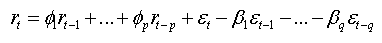(1)

Or equivalently in a compact form we have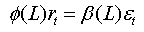Where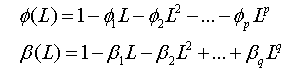If p and q are integers and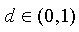we say that the time series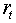is an ARIMA (p, d, q) series if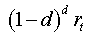is stationary. ARMA (p, q) time series is defined by the infinite sum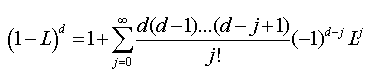(2)

Where the difference operator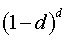.

If after the d differencing, we can represent the above equation as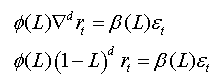Thus we have an ARIMA (p, d, q) model corresponding to an invertible ARMA (p, q) model after the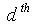differencing. An examination of ARIMA (p, d, q) model shows that it varies generally with different combinations of p and q. We can possibly generate a wide range of time series models, if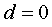, the ARIMA model reduces to an ARMA model and when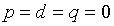, it becomes a pure white noise.

2.2. Volatility

Statistically, we can say it is the measure of dispersion of returns for a given security or market index. Volatility can either be measured by using the standard deviation or variance between returns from the same security or market index. Commonly, the higher the volatility, the riskier the security. As regards option pricing, it is described as a variable expressing the extent to which the return of the underlying asset will fluctuate between now and the expiration date. In other words, volatility refers to the amount of uncertainty of risk about the size changes in the value of an asset. The mathematical representation of volatility is given as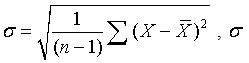represents the volatility, n represents the number of observed elements, X represents each observed element and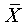represents the mean of all observed elements.

2.3. Data and Descriptive Statistic

The data employed in this study contains the demand and supply of electricity commodity in Nigeria. The figures span from 1970 to 2005 amounting to 36 data points. The choice of this dataset was limited to the availability of data especially the absence of monthly figures for demand and supply of electricity which is expected to give us high frequency. All the annual data series were obtained from the National Bureau of Statistics, Abuja, Nigeria.

Basically, it is a known fact that in nature, most economic series are non-stationary. We can therefore perform different types of transformation on the data series in order for us to make it stationary.

Let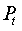denote value at time t and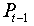the value at time t-1, and then the continuous compounded returns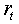are calculated from the corresponding first difference of the log of values expressed in percentage as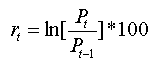(3)

Our choice of the compounded returns above is due to the fact that we wish to linearize the trend which definitely leads to a stable variance. The compounded returns also exhibit additive property which is not shared by relative returns. This better illustrates this property; we wish to use the theorem given below:

THEOREM 1 

If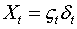, where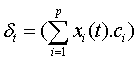Then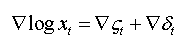is a stationary process.

Proof

The only term we considered is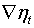. Differencing the function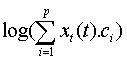This leads to the results: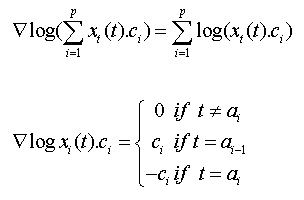Hence we have a zero difference except at the partition point where the result is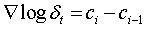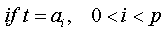.This completes the proof.

2.4. Tests for Stationarity

We wish to carry out checks on the respective return series which we obtained in order to find out if they are stationary or not. As stated above, this procedure is very much necessary due to the fact that no inference procedure can be applied to non-stationary series. Non-stationary series do not produce reliable estimates of parameters. We examined the graphs of both the demand-supply series and their return series with their respective Auto Correlation Form(ACF) and Partial Auto Correlation Form(PACF) to form a reasonable opinion on whether the series are stationary or not [6, 14].

2.5. Estimation of the GARCH (1,1) Model

The descriptive statistics and other properties of return series such as mean, variance, mean, median, kurtosis, skewness, standard deviation etc. have serious implications for modeling. This is due to the fact that they provide good decision for the distribution and process that drives it.

We shall estimate the parameters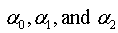of the GARCH (1,1) model using the Matlab. From the estimation we wish to obtain the market volatility. The GARCH model is given as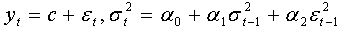Where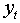Represents the returns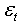Represents the error term (white noise)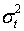Represents the variance at time t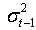Represents the variance at a previous time t-1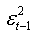Represents the error term at a previous time t-1

2.6. Derivation of the Electricity Demand and Supply Functions Using the Harvey Logistic Model

We are interested in developing a function for the demand of electricity commodity. Therefore we wish to use the Harvey Logistic Model [9, 11] given below;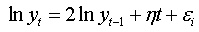(4)

Where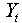is the electricity consumption in year t and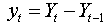for t=2…T.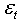is a disturbance term with zero mean and constant variance.

We shall therefore use ordinary least square method of regression to calibration the model.

Therefore,. Now let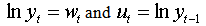Hence,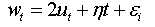(5)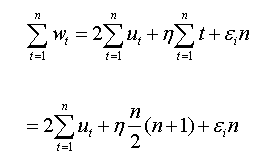(6)

If we multiply equation (5) by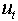, then we have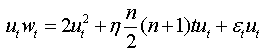(7)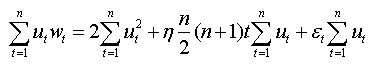(8)

We will use the ordinary least square method of regression  to obtain the disturbance or error term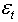and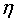.

The supply function formula can be obtaining from the equation below: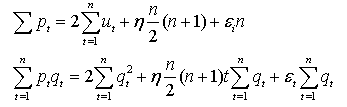### 3. Results

The data analyzed in this study consist of both the demand and supply available data for electricity for the Nigerian electricity market. Each data is represented by a series of 36 data points, so there are two different series with a total of 72 data points. Their time plots are presented in Figure 1. All computations and analysis are done using the matrix laboratory (MATLAB) software package and the statistical package for social sciences (SPSS).

Figure 1. Time plot of the yearly supply of electricity. Source: Nigerian Bureau of Statistics
3.1. Continuous Compounded Returns

We transformed the supply and demand series into returns series in order to eliminate the effects of the random variations detected in the plots of the supply and demand data series. The results obtained by the use of equation (1) are presented below:

In the demand and supply return time plots in Figure 1 and Figure 2 there is stationarity in the return series. The demand shows mean reversal property. It goes up and returns to the mean and goes down and returns to the mean. There are also spikes in the demand and supply because of data size which is small because of the information in the Table 1 and Table 2 were on annual basis which led to smaller spikes.

The noticeable feature of the two time series of returns suggests that fluctuations occurred at different amplitudes though very low amplitudes. There are periods of very short turbulence and longer period of relative calmness. This is due to the fact that our data series is very small. However, square return allows perfect stationarity. The Figure 5 is the time plot of supply squared returns and Figure 5 is the time plot of demand squared returns to allow the data to be stationary.

Table 2 provides the general overview and preliminary statistics of the respective returns for both data series obtained for the period under preview. These include the mean, minimum, maximum, standard deviation skewness and kurtosis. The large difference between the maximum and minimum is due to the fact that there are some levels of fluctuations in the returns. The mean and standard deviation of the two data series are significant. Both series are leptokurtic in nature although there is no indication of negative skewness due to the size of the data.

Using the SPSS software we obtained the following results:

#### Table 1. Descriptive statistics for the return series

Skew is a measure of heavy tails and asymmetry of a distribution (normal) and kurtosis is measure of too many observations around the mean for a distribution (normal).

The Matlab code for estimating the garch parameters is:

>> whos

>> y=price2ret (demand);

>> [coeff, errors, LLF, innovations, sigma, summary] =garchfit (y);

>> garchdisp (coeff, errors);

From the garch parameter code we obtained the results in the Table 2 and the Table 3 as follows:

#### Table 3. Parameter estimation of GARCH (1,1) for the Demand data series

From Table 1, we can therefore set up the GARCH (1,1) model with the appropriate estimated values: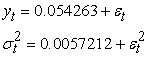From Table 3, we can therefore set up the GARCH (1, 1) model with the appropriate estimated values as follows: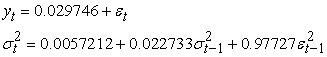From the above equation, we used the Monte Carlo simulation to obtain the white noisefrom the demand data series, from which we now obtained the variance to be 0.0066.

Hence our volatility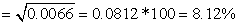.

3.2. Statistical Computation on Demand and Supply Variables

#### Table 5. Statistical table for Electricity Demand

We obtained the following from the supply data series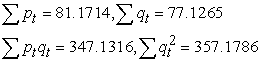We obtained the equations below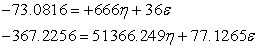In matrix form we have,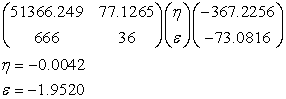The values of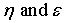for the demand and supply variables and plotting of graphs are obtained using the following Mat lab code in the appendix. Hence, the supply function is given as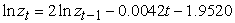We, therefore, obtained the following from the demand series.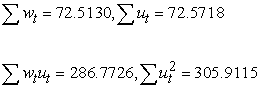We have two equations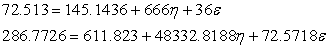Hence, in matrix form we have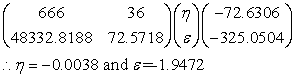The demand model is then given as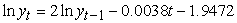The prediction for electricity demand and supply is obtained from the Harvey model by extrapolating the data from the year 2005 to 2026. The prediction value clearly indicts that the demand for electricity in Nigeria is continuously increasing and clearly outweighed the supply from Figure 10. Therefore, there are investment potentials for investors in the area of generation, transmission and distribution of electricity.

### 4. Conclusion

The National Electricity Regulation of Nigeria had suggested the minimum electricity requirement of Nigeria is about 50,000 Megawatts. At the moment the nation can boast of 4000 Megawatts, that is, a power shortfalls of about 46,000 Megawatts.1 kilowatt of electricity in Nigeria costs 13 naira and 165 naira is equivalent 1 US dollar. Therefore, the profit to be made if the shortfalls are supplied by new independent power stations, by simple calculation, is 317.48 billion US dollars. Therefore is vast window of opportunities open to investors in power section in Nigeria.

### Acknowledgements

The authors are grateful to the National Mathematical Centre, Abuja and The University of Abuja, Nigeria. The authors are grateful to the National Mathematical Centre, Abuja and The University of Abuja, Nigeria. The authors appreciate the suggestion of the reviewers which help to improve the quality of the paper.

### References

  Ackerman, G Short-Term Load Prediction for Electric-Utility Control of Generating Units. Comparative models for electrical Load forecasting, 1985, pp. 32-42, New York.In article  Al-Alawi, S.M and Syed, M.Islam, Principles of Electricity Demand Forecasting: Part 1 Methodologies. Power Engineering Journal 10 (3), 1996, 139-143.In article CrossRef  Al-Garni, A.Z., Syed, M.Z. and Javeed, S.N. (1994) A Regression Model for Electric-Energy-consumption forecasting in Eastern Saudi Arabia. Energy, 19 (10), 1994. 1043-1049.In article  Bilgin, M., Geopolitics of European Natural Gas Demand: Supplies from Russia, Caspian and Middle East. Energy Policy, 2009.In article CrossRef  Bamgbola M and Oke M Minimization of losses on electric power transmission line. Mathematical theory and modeling, Vol. 3, (7), 2013, 28-31.In article  Bassey B E Modeling volatility of the Nigerian Stock return using time series models (heteroscedasicity approach). M.sc dissertation submitted University of Abuja, Nigeria, 2009.In article  Bodger P.S and Tay, H.S ‘Logistic and energy substitution Models for Electricity Forecasting: A Comparison Using New Zealand Consumption Data’ Technological Forecasting and Social Change, Vol. 31, 1987, pp. 27-48.In article CrossRef  Dahl, C.A, A survey of Energy Demand Elasticities in Support of Development of the NEMS. US Department of Energy, 1993.In article  Dilaver, Z and Hunt, L.C., Industrial Electricity for Turkey: A Structural Time Series Analysis. Energy Economics 33, 2011, pp. 426-436.In article CrossRef  Dapice, D. “Electricity demand and supply in Myanmar”. Electric Power Research Institute, (1977) “Forecasting and Modeling Time-of-Day and Seasonal Electricity Demands” EPRI EA-578-SR, Palo Alto, CA. 2012.In article  Harvey, Andrew and Siem Jan Koopmans, “Forecasting Hourly Electricity Demands Using Time-Varying Splines,” Journal of the American Statistical Association 88, 1993 pp 1228-1237.In article CrossRef  North America Electric Reliability Council “Electricity Supply and Demand for 1990-1999”. 1990,Princeton New jerseyIn article  Jaimungal, S. IMPA Commodities course: Electricity Models, 2008. http://www.utstat.utoronto.ca/sjaimung/courses/Commodities/Electricity.pdfIn article  Oyelami, B O “Models for pricing of electricity commodity”. Mathematical Theory and modeling, Vol. 3 (1), 2013, 71-85.In article  Sambo, A, Garba, B, Zarma, H and Gaji, M, Electricity Generation and the present Challenges in the Nigerian Power sector. Technical paper Energy Commission of Nigeria, Abuja-Nigeria, 2009.In article  Solibakke, P.B., Describing the Nordic Forward electricity market: A stochastic model approach. International Journal of Business.11 (4), 2006, 345-349.In article

### Appendix

Matlab code for supply function parameters

>> Syms (‘x’, ‘y’)

>>% INPUT THE VALUE of companion matrix

>> A= [51366.2490 77.1265; 666 36]

A =1.0e+04 *5.1366 0.0077 0.0666 0.0036

>> % input the value of vector b

>> b= [-367.2256;-73.0816]

b = -367.2256-73.0816

>> % compute the inverse of matrix A

>> Inv (A)

Ans =0.0000 -0.0000-0.0004 0.0286

>> x=inv (A)*b

x =-0.0042-1.9520

Matlab code for demand function parameters

>> Syms (‘x’,‘y’)

>>% Input the value of the companion matrix A2

>> A2= [666 36; 48332.8188 72.5718]

A2 =1.0e+04 *0.0666 0.0036 4.8333 0.0073

>>% input the value of vector b2

>> b2= [-72.6306;-325.0504]

b2 =-72.6306-325.0504

>>% compute the inverse of A2

>> Inv (A2)

Ans =-0.0000 0.0000 0.0286 -0.0004

>> x=inv (A2)*b2

x =-0.0038-1.9472

>> t=1970:1:2013;

>>% import values of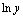and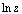.

>> plot (t, lnz)

>> plot (t, lny)

>> plot (t, lnz, t, lny)